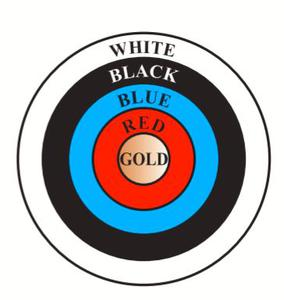GFG App
Open AppBrowser
Continue

# Class 10 NCERT Solutions – Chapter 12 Areas Related to Circles – Exercise 12.1

### Question 1. The radii of the two circles are 19 cm and 9 cm respectively. Find the radius of the circle which has a circumference equal to the sum of the circumferences of the two circles.

Solution:

Since we have to find the radius of the final circle we will use the formula of the circumference of circle C = 2πr.

Circumference of a circle with a radius of 19 cm is 2π * 19 = 38π cm.

Circumference of a circle with a radius of 9 cm is 2π * 9 = 18π cm.

Total Circumference is = 38π + 18π = 56π cm

Now using C = 2πr3  we will find r3 which is:

56π = 2π * (r3)

Therefore, the radius of the circle which has a circumference equal to the sum of the circumference of the given two circles is 28 cm.

### Question 2. The radii of the two circles are  8 cm and  6 cm respectively. Find the radius of the circle having an area equal to the sum of the areas of the two circles.

Solution:

Area of circle is = πr2 cm2

Area of circle1 = 64π cm2

Area of circle2 = 36π cm2

Total Area = 100π cm2

Now in order to find the radius of the required circle:

100π = πr2

r = 10 cm.

Therefore, the radius of the circle having an area equal to the sum of the areas of the two circles is 10cm.

### Question 3. The Figure depicts an archery target marked with its five scoring regions from the centre  outwards as Gold, Red, Blue, Black, and White. The diameter of the region representing the Gold score is 21 cm and each of the other bands is 10.5 cm wide. Find the area of each of the five scoring regions.Solution:

Radius (r1) of gold region (i.e., 1st circle) = 21/2 = 10.5 cm

Also, as per the question, it is Given that each circle is 10.5 cm wider than the previous circle.

Therefore, radius (r2) of 2nd circle = 10.5 + 10.5 = 21 cm

Radius (r3) of 3rd circle = 21 + 10.5 = 31.5 cm

Radius (r4) of 4th circle = 31.5 + 10.5 = 42 cm

Radius (r5) of 5th circle = 42 + 10.5 = 52.5 cm

Area of gold region = Area of 1st circle =  π*(10.5)*(10.5) cm2 = 346.5 cm2

Area of red region = Area of 2nd circle − Area of 1st circle  = π*(21)*(21) – π*(10.5)*(10.5) = 1039.5 cm2

Area of blue region = Area of 3rd circle − Area of 2nd circle =π*(31.5)*(31.5) -π*(21)*(21) = 1732.5 cm2

Area of black region = Area of 4th circle − Area of 3rd circle = π*(42)*(42)-π*(31.5)*(31.5)= 2425.5 cm2

Area of white region = Area of 5th circle − Area of 4th circle=π*(52.5)*(52.5)- π*(42)*(42)= 3118.5 cm2

Hence, areas of gold, red, blue, black, and white regions are 346.5 cm2, 1039.5 cm2, 1732.5 cm2, 2425.5 cm2, and 3118.5 cm2 respectively.

### Question 4. The wheels of a car are of diameter 80 cm each. How many complete revolutions does each wheel make in 10 minutes when the car is traveling at a speed of 66 km per hour?

Solution:

The diameter of the wheel of the car = 80 cm

So the Radius (r) of the wheel of the car = 40 cm

Now Calculating the Circumference of wheel = 2πr = 2π (40) = 80π cm

Speed of car = 66 km/hour

Speed(cm/min)= 110000 cm/min

Distance travelled by the car in 10 minutes = 110000 × 10 = 1100000 cm

Let the number of revolutions of the wheel of the car be p.

So, equating it now

We get, p × Distance traveled in 1 revolution = Distance traveled in 10 minutes

80π * p = 1100000

p = 4375

Hence, each wheel of the car will make 4375 revolutions.

### (A) 2 units (B) π units (C) 4 units (D) 7 units

Solution:

Let the radius of the circle be r.

Circumference of circle = 2πr

Area of circle = πr2

Also in the question, it is given that, the circumference of the circle and the area of the circle are equal.

Hence, 2πr = πr2

r = 2 units

Hence, the radius of the circle is 2 units.

Option A is the correct answer.

My Personal Notes arrow_drop_up